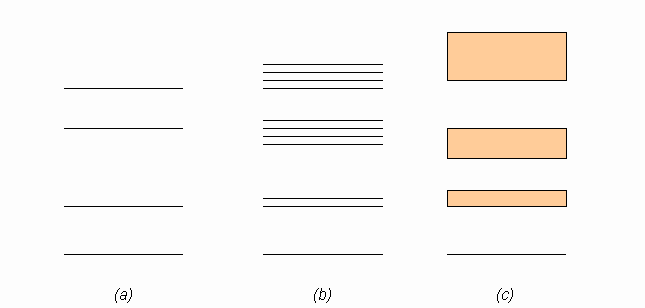# A problem with a figure related to band theory

• I
• DaTario
In summary, the conversation discusses a diagram showing the introduction of band theory in solid state physics. It is explained that the diagram is a common way of visualizing the concept, with part (a) representing single atom states and part (b) representing multiple atoms in a lattice. However, there is concern about the accuracy of part (b) as it appears to show a mix of twofold and fourfold splitting, and suggests that the ground state does not suffer any splitting.

#### DaTario

TL;DR Summary
Hi, All, I would like to know the following figure is ok in the context of explaining to beginers the theory of bands (solid state physics).
Hi, All. In searching for images related to the introduction of band theory in solid sate physics I found this one:I would like to ask you if I am correct in thinking the increase in the number of splittings (in b) is misleading to students.

Thank you all
Best regards,
DaTario

Last edited:
First: where did you get this diagram?

Ok, now on to an explanation:
Part (a) looks like a set of single atom states.
Part (b) is for multiple atoms in a lattice, looks like two.
Part (c) is for a many atom lattice

These kind of drawings are quite common and I've seen them in several books. The "idea" is that you start with a single atom and you have the standard atom energy levels (drawing a). With two close atoms we must split the atom levels to satify Pauli's principle (the splitting is shown very large to ease reading, drawing b). You keep adding many, many atoms until the discrete energy levels split in so many levels that the system looks like a continuum (drawing c). Kittel's or Ashcroft's books take this approach to introduce the subject

•hutchphd
Dr Transport said:
First: where did you get this diagram?

I got it from image googling with key word "band theory solid state physics".

It seems that (b) shows neither a twofold splitting nor a fourfold splitting. So it seemed confusing to me.

Gordianus said:
These kind of drawings are quite common and I've seen them in several books. The "idea" is that you start with a single atom and you have the standard atom energy levels (drawing a). With two close atoms we must split the atom levels to satify Pauli's principle (the splitting is shown very large to ease reading, drawing b). You keep adding many, many atoms until the discrete energy levels split in so many levels that the system looks like a continuum (drawing c). Kittel's or Ashcroft's books take this approach to introduce the subject
I understand it. I am worried with the drawings in (b), because it seems to show in one figure a mix of a twofold and a fourfold splitting.

It also bothered me that the figure also suggests that the ground state does not suffer any kind of split.

It does indeed look wrong, each single particle state should split into as many states as there are particles in the system.

•DaTario